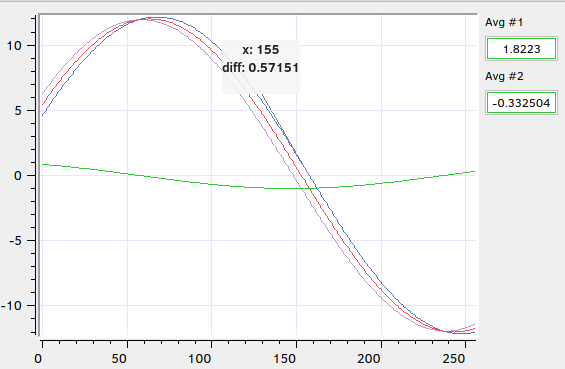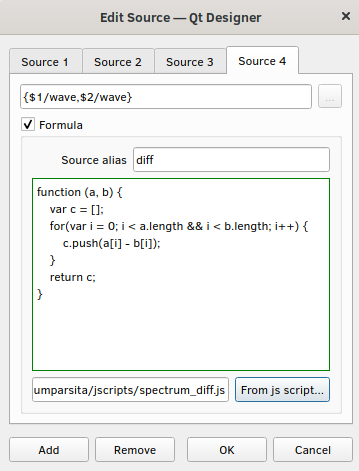cumbia formula plugin  4.x

The CuFormulaPlugin class

# Introduction

CuFormulaPlugin lets you employ sources in the form of javascript functions, rather than simple variable names exported by the control system(s) in use.

# Usage

The application must first load the formula plugin. Once loaded, the CuFormulaPlugin::initialize method must be called passing a pointer to a previously allocated CumbiaPool and a reference to a CuControlsFactoryPool. The CuFormulaPlugin::initialize function takes care of registering the default "formula://" domain in CumbiaPool and CuControlsFactoryPool, by calling CumbiaPool::registerImpl and CuControlsFactoryPool::setSrcPatterns

// cumbia
#include <cumbiapool.h>
#include <quapps.h>
// formula plugin
#include <cuformulaplugininterface.h>
MyApp::MyApp(CumbiaPool *cumbia_pool, QWidget *parent) :
QWidget(parent)
{
// module loader first: initialize cumbia pool and factory pool
QObject *o;
CuFormulaPluginI *formula_p = pl.get<CuFormulaPluginI>("cuformula-plugin.so", &o);
if(formula_p) { // ok
formula_p->initialize(cumbia_pool, m_ctrl_factory_pool);
ui = new Ui::MyApp; // create and setup ui
ui->setupUi(this, cu_pool, m_ctrl_factory_pool);
// ...
}
2. formula source syntax

As suggested by the discusssion above, in order to be interpreted by the plugin a source must start with the "formula://" pattern. The complete source expression will then contain the list of control system source names within curly braces, comma separated, and a javascript function expressing the relations among the sources:

QString src = "formula://{a/test/device/att,b/test/device/att} function(a, b) { return a + b }"
my_sum_label->setSource(src); // let my_sum_label be a QuLabel

The result of the formula specified in the code will be displayed in the label.

Example. la-cumparsita

The image below shows formulas applied to a couple of waveforms generated by the TangoTest device. You can see the sum and diff curves, calculated by javascript functions from the readings of the two wave attributes. On the right, two QuLabel show the average value of the waves for each of the two sources.Figure 1. la-cumparsita shows a sum and a difference curve. Average labels on the right
3. Formulas in the Qt Designer

As every other source, a formula can be set directly from the Qt designer form editor. Right click on any cumbia-qtcontrols widget and select the Edit connection... action. In the popup dialog, check the Formula box and write the JavaScript function in the text area below. The function can be also loaded from a file: click on the From js script... button and choose the input file. The contents of the file are copied into the text area and when the "OK" button is clicked they become the source of the control widget. If one provides a Source alias in the dedicated text field, it may be used as an alias for the formula, for example as a title of a plot curve (see figure above). The input parameters to the function, if provided, are replaced by the sources listed in curly braces in the top text line edit (see figure below).

Note
The contents of the javascript file are copied into the ui file, so that the js file does not need to be part of the project. On the other hand, changing the js file alone is not enough to apply the changes back in the source: the file must be reloaded through the Qt designer Edit connection... dialog.Figure 2. Edit source dialog with a javascript function

In the example above, the readings from the two sources

• \$1/wave and
• \$2/wave

will replace the a,b input parameters in the Javascript function. A third vector, named c in the function, is returned and used to provide data for the Source 4, that's been given the diff alias (as in Figure 1).